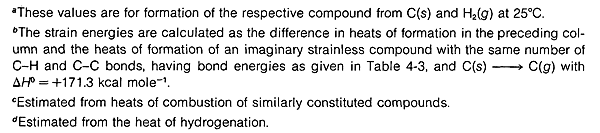# 12.10: Strain in Polycyclic Molecules

Knowing the importance of angle and eclipsing strain in the small-ring cycloalkanes, we should expect that these strains would become still more important in going from cyclobutane to bicyclo[1.1.0]butane or from cyclooctane to pentacyclo[4.2.0.0$$^{2.5}$$.0$$^{3.8}$$.0$$^{4.7}$$]octane (cubane). This expectation is borne out by the data in Table 12-6, which gives the properties of several illustrative small-ring polycyclic molecules that have been synthesized only in recent years.

The extraordinary strain energy of cubane $$\left( \sim 142 \: \text{kcal mol}^{-1} \right)$$ is worthy of special note. It is roughly equal to six times the strain energy of a single cyclobutane ring $$\left( \sim 26 \: \text{kcal mol}^{-1} \right)$$ as befits a molecule made up of six cyclobutane rings as faces. Despite this, cubane is surprisingly stable to spontaneous decomposition processes, although it will rearrange under the influence of metal or acid catalysts.

Another extraordinarily strained polycyclic hydrocarbon that has been prepared is prismane (the Ladenburg structure for benzene).

This substance is a liquid that decomposes explosively when heated. In dilute solution at $$100^\text{o}$$, it is converted slowly to benzene.

One of the most interesting types of polycyclic carbon compounds prepared in recent years is the group of tricyclic substances known as “propellanes.” A typical example is tricyclo [3.2.2.0$$^{1.5}$$]nonane, which sometimes is called [3.2.2]propellane, $$12$$. The physical properties of several of these are included in Table 12-6. A quick look at formula $$12$$ probably does not suggest any great structural difference from the bicyclic compounds we have discussed previously. However, if one tries to construct a ball-and-stick model of $$12$$, one soon concludes that the propellanes are truly extraordinary substances in that all four carbon bonds at the bridgehead carbons extend, not to the comers of a tetrahedron, or even a distorted tetrahedron as for a cyclopropane ring, but away from the carbon on the same side of a plane through the carbon as in $$13$$:

Table 12-6: Properties of Some Small-Ring Polycyclic HydrocarbonsAngle strain is severe. Accordingly, [3.2.1]propellane reacts rapidly with bromine at $$-60^\text{o}$$ and with hydrogen over palladium at room temperature:Still another way to torture the valence angles of carbon is to twist a double bond by connecting it to the bridgehead carbon of a bicyclic system with reasonably small rings, as in bicyclo[3.3.1]-1-nonene, $$14$$:As with $$12$$, it might appear that there is nothing unusual about $$14$$. But a ball-and-stick model of $$14$$ reveals that the carbon-carbon double bond is in a strained configuration like $$15$$. Some of the properties of $$14$$ are given in Table 12-6. That compounds with a double bond to a bridgehead carbon, such as $$14$$, should be highly strained is known as “Bredt’s Rule.” The most spectacular example of this form of molecular distortion reported so far is bicyclo[2.2.1]-1-heptene, $$16$$, for which evidence has been adduced that it is an unstable reaction intermediate: